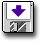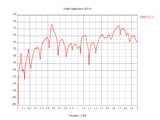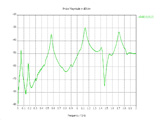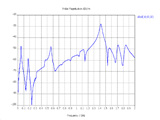Modeling Powerbus and Cable with CST

 Geometry and setup Double-sided PCB: Size: 125 mm *100 mm *1 mm Top and bottom metal: PEC Dielectric: FR4, εr = 4.5, loss tangent = 0.015 @ 1 GHz (Specification: Const. fit tan delta) Cable (flat ribbon): Length: 1000 mm Width: 8 mm Material: PEC Model parameters: Frequency: 5 MHz - 2 GHz Steady state accuracy limit: -40 dB Mesh definition: Mesh type: Hexahedral Mesh density control: Lines per wavelength = 40, Others = Default Special mesh properties: Refine at PEC/lossy metal edges by factor = 6, Others = Default Automesh Excitation: Discrete port (1 V, 50 ohms)cst_powerbus_cable.zip Simulation result Simulation time: 10 hrs, 19 mins, 24 secs Number of mesh cells: 8012970 Excitation duration: 3.563457e+000 ns Calculation time for excitation: 4717 secs Number of calculated pulse widths: 17.0145 Simulated number of time steps: 53789 Maximum number of time steps: 63227 Decisions the user must make that affect the accuracy of the result Mesh density control: the minimum Lines per wavelength is 40. Special mesh properties: 6 or greater value is needed for 'Refine at PEC/lossy metal edges by factor' to obtain higher accuracy. Boundary: 'Automatic minimum distance to structure' is set to 2th part of wave. Low frequency: a separate model focused on low frequencies is created to replace the low frequency part (5 MHz - 300 MHz). Comments What source do we use in this example? In this example, we use 'Voltage' port type with voltage of 1.0 volts connected with a 50 ohms impedance in series to get the electric field. Can CST model dielectric materials with constant loss tangent?CST cannot model materials with constant loss tangent. In this model, we model the dielectrics having a loss tangent of 0.015 at 1 GHz and select the specification of 'Const. fit tan delta'. See the comment in the second example to get more information... How is the cable modeled? A flat PEC ribbon instead of round cable is used to model the cable. The width of the cable is 8 mm (4 times the radius of the cable). This substitution will decrease the simulation time without sacrificing accuracy. more information... How to get the accurate results at low frequencies? At low frequencies, a large boundary is needed. Thus a separate model focused on low frequencies is created to replace the low frequency part (5 MHz - 300 MHz). In this sub model, 'Automatic minimum distance to structure' is set to 2th part of wave at center frequency between 5 MHz to 300 MHz. This means the boundary is expanded compared to that at high frequency range. Correspondingly, the finer mesh is needed to make the model calculable. 'Lines per wavelength' is set to 50; 'Refine at PEC/lossy metal edges by factor' is set to 6, 'Mesh line ratio limit' is set to 80; 'Steady state accuracy limit' is set to -50 dB. It took another 3 hours to solve this sub model for low frequency part.
 Screen shotsFig. 3. Electric field at 10 m, theta=0o, phi=0oFig. 4. Electric field at 10 m, theta=90o, phi=0oFig. 5. Electric field at 10 m, theta=90o, phi=90o